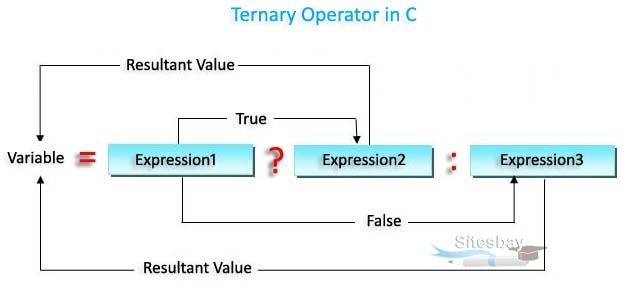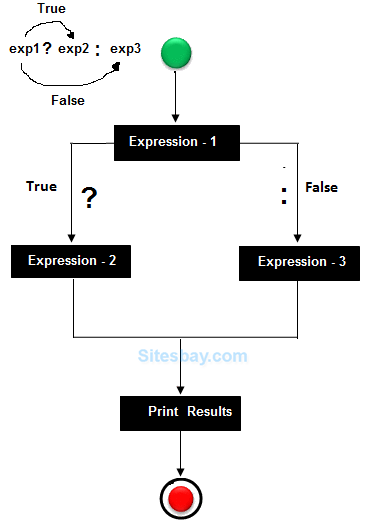# Ternary Operator in C

## Ternary Operator in C

If any operator is used on three operands or variable is known as Ternary Operator. It can be represented with ? : . It is also called as conditional operator

It helps to think of the ternary operator as a shorthand way or writing an if-else statement. Programmers use the ternary operator for decision making in place of longer if and else conditional statements.

The ternary operator is an operator that takes three arguments. The first argument is a comparison argument, the second is the result upon a true comparison, and the third is the result upon a false comparison. Ternary operator is shortened way of writing an if-else statement.

Ternary operator is a?b:c it say that the condition a is true b will be executed else c will be executed.Using ?: reduce the number of line codes and improve the performance of application.

## Syntax

```expression-1 ? expression-2 : expression-3
```In the above symbol expression-1 is condition and expression-2 and expression-3 will be either value or variable or statement or any mathematical expression. If condition will be true expression-2 will be execute otherwise expression-3 will be executed.

## Syntax

```a<b ? printf("a is less") : printf("a is greater");
```

## Flow Diagram## Find Largest Number among 3 Number in C

```#include<stdio.h>
#include<conio.h>

void main()
{
int a, b, c, large;
clrscr();
printf("Enter any three number: ");
scanf("%d%d%d",&a,&b,&c);
large=a>b ? (a>c?a:c) : (b>c?b:c);
printf("Largest Number is: %d",large);
getch();
}
```

## Output

```Enter any three number: 5 7 2
Largest number is 7
```

## Example for Ternary Operator in C

```#include<stdio.h>
int main()
{
int a=2,b=4,c=9;
int lar;
lar=(((a>b)&&(a>c))?a:((b>c)?b:c));
printf(“Largest Number is: %d”,lar);
return 0;
}
```

## Output

```Largest Number is: 9
```